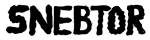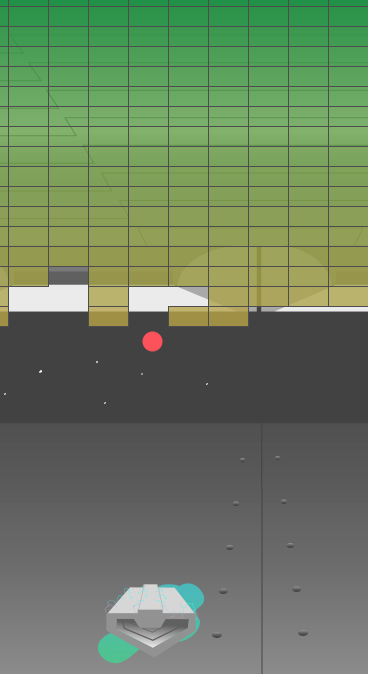### Arkanoid clone

processing``` /** * a arkanoid clone by http://www.local-guru.net * use the mouse to play```
``` slightly modyfied by snebtor.org Replacing graphic elements with pshape */ int px, py; int vx, vy; PShape ship; PImage back; boolean paused = true; boolean done = true; int[][] stones; void setup() { //noCursor(); size(1280,720); ship = loadShape("ship3.svg");// vector loader back=loadImage("bg.png");//raster loader smooth(); px = width/2; //position of ball x py = height-70;//initial position of ball y vx = int(random( -25, 25 ));//initial speed vy = -40; stones = new int; //two dimesnional array of stones for( int x = 0; x < 31; x++) { for( int y = 0; y < 17; y++ ) { stones[x][y] = y + 1; } } textFont( loadFont( "font.vlw" )); } void draw() { //shape(bg,0,0);// needs to be a simple image in this case we rasterize background(back); stroke(60); strokeWeight(1); noCursor(); // update postion of the ball if (!paused) update(); // draw all stones that are not removed yet // check if all are gone done = true; for( int x = 0; x < 31; x++) { for( int y = 0; y < 17; y++ ) { //create new rows or columns of elements if ( stones[x][y] > 0 ) { done = false; fill( 1 + 10 * stones[x][y],145,52,200 );//this is an array for the gradient fill rect( 10 + x * 40, 10 + y * 20, 40, 20 ); //this is the stone design } } } // no stone remaining - display yippie message if ( done ) { paused = true; fill(255); textSize( 48 ); text( "JIPPIE!", 50, 200 ); } // display text if paused if ( paused ) { textSize( 16 ); fill(128); text( "press mousebutton to continue", 10, 650 ); } //draw starts here // draw paddle shape( ship, mouseX+106, height-280, ship.width/3, ship.height/3 ); // here we locate the new vector file considering width of graphic fill(mouseX,56,70); noStroke(); // draw ball ellipse(px,py,20,20); } void update() { // check if ball dropped out of the lower border if ( py + vy > height - 10 ) { px = width/2; py = height-70; // make the distances relative to size of the screen vx = int(random( -40, 40 )); //change speed here too vy = -19; paused = true; } // check if the ball hits a block for( int x = 0; x < 31; x++) { for( int y = 0; y < 17; y++ ) { if ( stones[x][y] > 0 ) { if ( px + vx + 10 > 10 + x * 40 && px + vx - 10 < 10 + x * 40 + 40 && py + vy + 10 > 10 + y * 20 && py + vy - 10 < 10 + y * 20 + 20 ) { stones[x][y] = 0; // change the velocity in y direction if the block has been hit // on the bottom or on the top if ( px + 10 > 10 + x * 40 && px - 10 < 10 + x * 40 + 40 ) vy = -vy; // change the velocity in the x direction if the block has been hit on the side if ( py + 10 > 10 + y * 20 && py - 10 < 10 + y * 20 + 20 ) vx = -vx; } } } } // change the direction if the ball hits a wall if (px + vx < 10 || px + vx > width - 10) { vx = -vx; } if (py + vy < 10 || py + vy > height - 10) { vy = -vy; } ```
``` // check if the paddle was hit // change the place where you want the ball to be matched with mouseX // take into consideration the height of your vector file if ( py + vy >= height-80 && px >= mouseX - 53 && px <= mouseX +53 ) { //change these values to map boundaries of ship, need to know size of ship vy = -vy; vx = int(map( px - mouseX, -33, 33, -18, 18 )); } // calculate new postion px += vx; py += vy; } void mousePressed() { paused = !paused; if (done) { for( int x = 0; x < 31; x++) { for( int y = 0; y < 17; y++ ) { stones[x][y] = y + 1; } } done = false; px = width/2; py = height/2; vx = int(random( -40, 40 )); vy = -18; } } ```